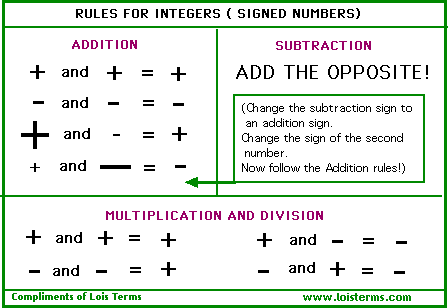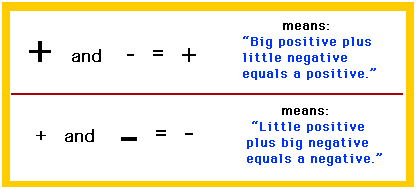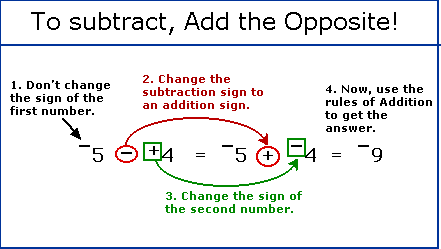Dear Lois: Please tell me the rules on how to add & subtract fractions in integer.Kimmie

Dear Kimmie: Thanks for your question. I am guessing that you want to know the rules for adding and subtracting fractions with positive and negative signs.

First of all, if you want to know about adding fractions, check out "How to Add Fractions". Be sure to follow the math examples carefully. To subtract fractions, follow the same steps as for adding, except subtract where you would add.

Now about those positive and negative signs. The rules are the same whether you are working with integers or fractions. I will give you a method of learning the rules for the signs that has worked for many other students.

Copy off this "Rules for Integers" chart and paste it on a large index card. Put the card in your math book or folder and refer to it often while you are doing your homework. If you keep using the card, you will get better with the signs.Notice how the Integer Rules chart works.

If both numbers have positive signs, then the answer will be positive.

`+   and    +   =    +            +3  +  +4  =  +7`

If both numbers have negative signs, then the answer will be negative.

`-  and   -  =   -          -3  +  -4  =  -7`

Suppose, however, you are trying to add numbers with different signs...which is the way life usually goes. What do you do then?When you are adding numbers with different signs, pretend that the numbers are arm wrestling. The two numbers step into the ring, let's say, +8 and -3. The numbers take off their robes--that is, their positive and negative signs. (Your teacher may call taking off the signs "absolute value.") The numbers both put their elbows on the table and go hand to hand, muscle to muscle. The big number always wins. In this case, 8 wins, because without its sign, 8 is bigger than 3. 8 wins the match by 5 points. (8 - 3 = 5). After 8 wins, its positive sign goes with 5. So, +8 + -3 = + 5.

Notice how the rules for adding numbers with different signs are shown on the chart:SUBTRACTION

There is just one simple rule for subtracting signed numbers.

Who likes to subtract? Nobody, that's who! I mean, subtracting is right up there at the top of the list of all-time, least favorite ways to spend an afternoon. So, why subtract when you can add?

Study the example below to see how to change a subtraction problem into addition. (1) Leave the sign of the first number alone. (2) Change the subtraction sign to an addition sign. (3) Change the sign of the second number. Changing the sign of the second number changes it to its opposite. (4) Now go back and follow the addition rules given above. Get it? "ADD THE OPPOSITE!"MULTIPLICATION AND DIVISION

You didn't ask for the multplication and division rules, Kimmie, but as an extra bonus, I am going to include them on the chart. If you're having to learn the addition and subtraction rules...well, it's only a matter of time.

The rules are the same for both multiplication and division. They make the rules for addition and subtraction look like a piece of cake. So here they are, pure and simple:

If the signs are alike, the answer will be positive.
If the signs are different, the answer will be negative.

`+4    x    +  3  =  +12-2    x    -  5  =  +10+7    x    -  2  =  -14-8    x    +  4  =  -32`

There, now isn't that easy?Hey, Students. Test you knowledge of the rules for integers. Take a Quickie Quiz!